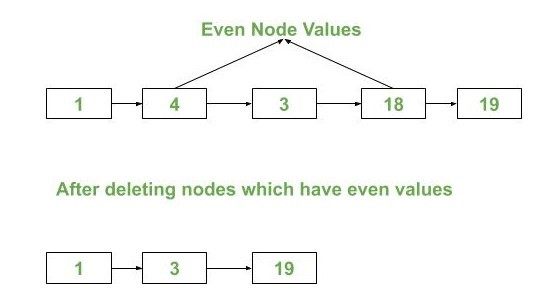# Program to delete all even nodes from a Singly Linked List

Given a singly linked list containing N nodes, the task is to delete all the even nodes from the list.Examples:

Input: LL = 1 -> 4 -> 3 -> 18 -> 19
Output: 1 -> 3 -> 19

Input: LL = 5 -> 3 -> 6 -> 8 -> 4 -> 1 -> 2 -> 9
Output: 5 -> 3 -> 1 -> 9

Approach:

• The idea is to traverse the nodes of the singly linked list one by one and get the pointer of the nodes having even data. Delete those nodes by following the approach used in this post.

Below is the implementation of the above idea:

 `// C++ implementation to delete all ` `// even nodes from the singly linked list ` ` `  `#include ` `using` `namespace` `std; ` ` `  `// Node of the singly linked list ` `struct` `Node { ` `    ``int` `data; ` `    ``struct` `Node* next; ` `}; ` ` `  `// Function to insert a node at ` `// the beginning of the singly ` `// Linked List ` `void` `push(``struct` `Node** head_ref, ` `          ``int` `new_data) ` `{ ` `    ``struct` `Node* new_node ` `        ``= (``struct` `Node*)``malloc``( ` `            ``sizeof``( ` `                ``struct` `Node)); ` ` `  `    ``new_node->data = new_data; ` `    ``new_node->next = (*head_ref); ` `    ``(*head_ref) = new_node; ` `} ` ` `  `// Function to delete a node in a ` `// singly Linked List. ` `// head_ref --> Pointer to head ` `// node pointer. ` `// key --> Node to be deleted ` `void` `deleteNode(``struct` `Node** head_ref, ` `                ``int` `key) ` `{ ` `    ``// Store head node ` `    ``struct` `Node *temp = *head_ref, ` `                ``*prev; ` ` `  `    ``// If head node itself holds ` `    ``// the key to be deleted ` `    ``if` `(temp != NULL ` `        ``&& temp->data == key) { ` `        ``// Changed head ` `        ``*head_ref = temp->next; ` `        ``// Free old head ` `        ``free``(temp); ` `        ``return``; ` `    ``} ` ` `  `    ``// Search for the key to be ` `    ``// deleted, keep track of the ` `    ``// previous node as we need ` `    ``// to change 'prev->next' ` `    ``while` `(temp != NULL ` `           ``&& temp->data != key) { ` `        ``prev = temp; ` `        ``temp = temp->next; ` `    ``} ` ` `  `    ``// If key was not present ` `    ``// in linked list ` `    ``if` `(temp == NULL) ` `        ``return``; ` ` `  `    ``// Unlink the node from ` `    ``// linked list ` `    ``prev->next = temp->next; ` ` `  `    ``// Free memory ` `    ``free``(temp); ` `} ` ` `  `// Function to delete all the ` `// even nodes from the ` `// singly linked list ` `void` `deleteEvenNodes(Node** head_ref) ` `{ ` `    ``Node* ptr = *head_ref; ` `    ``// Node* next; ` ` `  `    ``while` `(ptr != NULL) { ` `        ``// next = ptr->next; ` `        ``// If true, delete node 'ptr' ` `        ``if` `(ptr->data % 2 == 0) ` `            ``deleteNode(head_ref, ` `                       ``ptr->data); ` `        ``ptr = ptr->next; ` `    ``} ` `} ` ` `  `// This function prints contents ` `// of linked list starting from ` `// the given node ` `void` `printList(``struct` `Node* node) ` `{ ` `    ``while` `(node != NULL) { ` `        ``printf``(``" %d -> "``, node->data); ` `        ``node = node->next; ` `    ``} ` `} ` ` `  `// Driver code ` `int` `main() ` `{ ` `    ``// Start with the empty list ` `    ``Node* head = NULL; ` `    ``push(&head, 19); ` `    ``push(&head, 18); ` `    ``push(&head, 3); ` `    ``push(&head, 4); ` `    ``push(&head, 1); ` ` `  `    ``printf``(``"Initial List: "``); ` `    ``printList(head); ` ` `  `    ``deleteEvenNodes(&head); ` ` `  `    ``printf``(``"\nFinal List: "``); ` `    ``printList(head); ` `} `

Output:

```Initial List:  1 ->  4 ->  3 ->  18 ->  19 ->
Final List:  1 ->  3 ->  19 ->
```

Time Complexity: O(N), where N is the total number of nodes.

Don’t stop now and take your learning to the next level. Learn all the important concepts of Data Structures and Algorithms with the help of the most trusted course: DSA Self Paced. Become industry ready at a student-friendly price.

My Personal Notes arrow_drop_upCheck out this Author's contributed articles.

If you like GeeksforGeeks and would like to contribute, you can also write an article using contribute.geeksforgeeks.org or mail your article to contribute@geeksforgeeks.org. See your article appearing on the GeeksforGeeks main page and help other Geeks.

Please Improve this article if you find anything incorrect by clicking on the "Improve Article" button below.

1

Please write to us at contribute@geeksforgeeks.org to report any issue with the above content.